Function Repository Resource:

# RandomPolynomial

Generate a polynomial with random terms

Contributed by: Jon McLoone
 ResourceFunction["RandomPolynomial"][var] generates a random polynomial in var with maximum degree 5. ResourceFunction["RandomPolynomial"][var,maxdegree] generates a random polynomial in var with maximum degree maxdegree. ResourceFunction["RandomPolynomial"][varlist,maxdegree] generates a random polynomial with maximum degree maxdegree in each of the elements of the varlist. ResourceFunction["RandomPolynomial"][varlist,degreelist] generates a random polynomial each of the elements of the varlist with degree chosen from degreelist. ResourceFunction["RandomPolynomial"][var,degree,termcount] generates a random polynomial with the number of terms given by termcount.

## Details and Options

The following option is supported:
 "TermMultiplierDistribution" the distribution to draw term multipliers from DiscreteUniformDistribution[{-100,100}]

## Examples

### Basic Examples (2)

Generate a random polynomial in x:

 In:=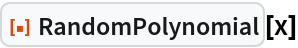Out=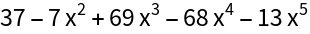Generate a random quadratic in x:

 In:=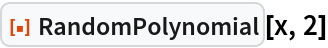Out=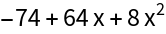Generate a random polynomial in x of maximum degree 100:

 In:=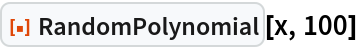Out=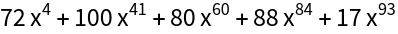Generate a random polynomial in x of maximum degree 100 with 3 terms:

 In:=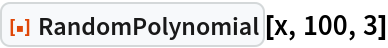Out=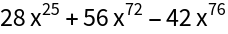### Scope (3)

Restrict to only even powers of x:

 In:=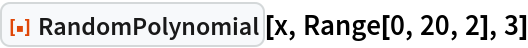Out=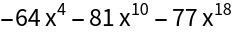RandomPolynomial can generate a polynomial in multiple variables:

 In:=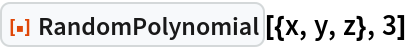Out=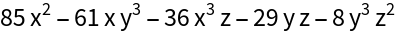RandomPolynomial can generate polynomials in any kind of expression, not just symbols:

 In:=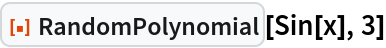Out=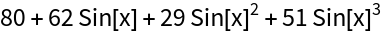Generate a Laurent polynomial by including negative order terms:

 In:=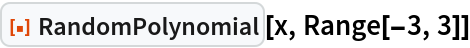Out=### Options (2)

#### TermMultiplierDistribution (2)

Generate a polynomial whose coefficients are integers in the range [0,100]:

 In:=Out=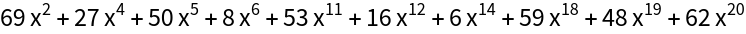Generate a polynomial with normally-distributed coefficients:

 In:=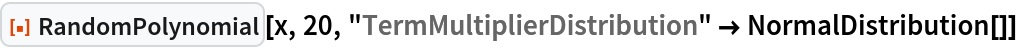Out=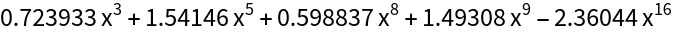### Possible Issues (1)

Requesting more terms than are possible causes a failure:

 In:=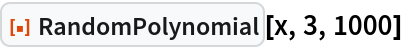Out=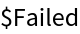Jon McLoone

## Version History

• 1.0.0 – 06 April 2022